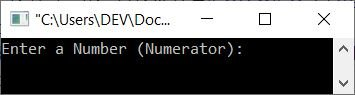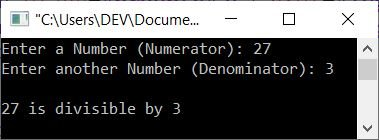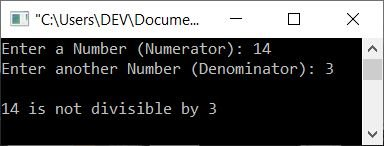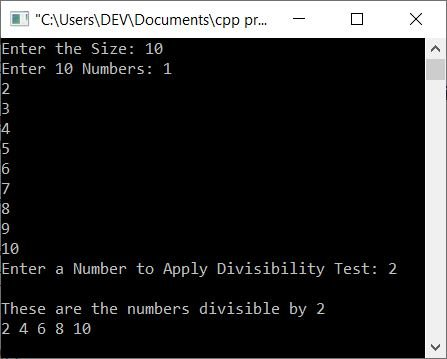# C++ program to check whether a number is divisible by another number

This article provides some programs in C++ that are used to check whether a number (entered by the user) is divisible by another number (also entered by the user) or not. These are the programs included:

## Using if-else logic, check if a number is divisible by another

The question is, "Write a C++ program that receives two numbers as input and checks whether the second number divides first without leaving any remainder or not." The answer to this question is the program given below:

```#include<iostream>

using namespace std;
int main()
{
int numerator, denominator;
cout<<"Enter a Number (Numerator): ";
cin>>numerator;
cout<<"Enter another Number (Denominator): ";
cin>>denominator;
if(numerator%denominator==0)
cout<<endl<<numerator<<" is divisible by "<<denominator;
else
cout<<endl<<numerator<<" is not divisible by "<<denominator;
cout<<endl;
return 0;
}```

The snapshot given below shows the initial output produced by the above C++ program on checking whether a number (entered by the user) is divisible by another number (also entered by the user) or not:Now enter any two numbers as the numerator and denominator to check whether the denominator completely divides the numerator (without leaving any remainder, or leaving 0 as the remainder) or not. For example, here is its sample run with user input 27 as numerator and 3 as denominator:Note: The % (modulo) operator gives the remainder. For example, 10%3 gives 1, 20%10 gives 0, and 20%7 gives 6.

Therefore, if numerator%denominator gives 0, it means that the denominator divides the numerator without leaving a remainder.

### Using a a user-defined function, check if a number is divisible by another

This is the same program as the previous one. That is, this program does the same job of checking whether the first number divides the second number or not. Both numbers must be entered by the user during program execution:

```#include<iostream>

using namespace std;

int check_div(int, int);
int main()
{
int numerator, denominator, res;
cout<<"Enter a Number (Numerator): ";
cin>>numerator;
cout<<"Enter another Number (Denominator): ";
cin>>denominator;

res = check_div(numerator, denominator);
if(res==1)
cout<<endl<<numerator<<" is divisible by "<<denominator;
else
cout<<endl<<numerator<<" is not divisible by "<<denominator;
cout<<endl;
return 0;
}
int check_div(int a, int b)
{
if(a%b==0)
return 1;
else
return 0;
}```

Here is its sample run with user input of 14 as the numerator and 4 as the denominator:The function check_div() in the preceding program returns 1 if a%b or numerator%denominator returns 0. Otherwise, it returns 0. Based on the return value, the print statement gets performed as shown in the above program.

### Using class, check if a number is divisible by another

This is another program that performs the same function as the previous two, but in a different way. That is, this program uses classes and objects, an object-oriented feature of C++.

```#include<iostream>

using namespace std;

class CodesCracker
{
public:
int check_div(int, int);
};
int CodesCracker::check_div(int a, int b)
{
if(a%b==0)
return 1;
else
return 0;
}
int main()
{
CodesCracker ob;
int numerator, denominator, res;
cout<<"Enter a Number (Numerator): ";
cin>>numerator;
cout<<"Enter another Number (Denominator): ";
cin>>denominator;

res = ob.check_div(numerator, denominator);
if(res==1)
cout<<endl<<numerator<<" is divisible by "<<denominator;
else
cout<<endl<<numerator<<" is not divisible by "<<denominator;
cout<<endl;
return 0;
}```

This program produces the same output as the previous program.

## Divisibility Test with Multiple Numbers in a Range

The question is: write a program in C++ that finds and prints all the numbers in a given range that are divisible by a given number (entered by the user). The program given below is the answer to this question:

```#include<iostream>

using namespace std;
int main()
{
int tot, i, arr, num, j, arr_div;
cout<<"Enter the Size: ";
cin>>tot;
cout<<"Enter "<<tot<<" Numbers: ";
for(i=0; i<tot; i++)
cin>>arr[i];
cout<<"Enter a Number to Apply Divisibility Test: ";
cin>>num;
for(i=0, j=0; i<tot; i++)
{
if(arr[i]%num==0)
{
arr_div[j] = arr[i];
j++;
}
}
if(j==0)
cout<<"\nAll the numbers are not divisible by "<<num;
else
{
cout<<"\nThese are the numbers divisible by "<<num<<endl;
for(i=0; i<j; i++)
cout<<arr_div[i]<<" ";

}
cout<<endl;
return 0;
}```

Here is its sample run with user input: 10 as size, 1, 2, 3, 4, 5, 6, 7, 8, 9, 10 as 10 numbers, 2 as the number to apply the divisibility test with all these given 10 numbers as shown in the snapshot given below:C++ Quiz

« Previous Program Next Program »